# Bond length and measurements of radius

22. Oct 2017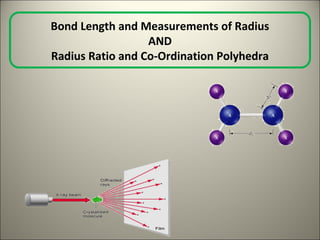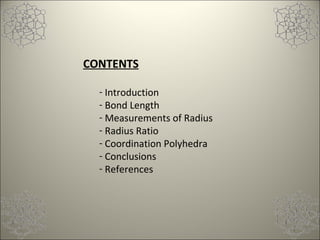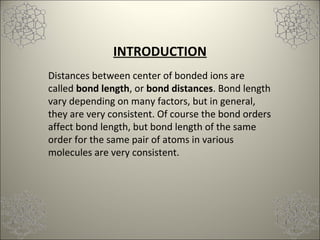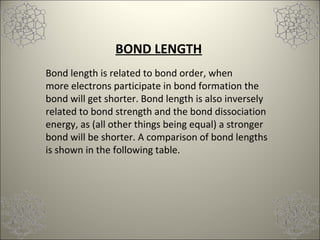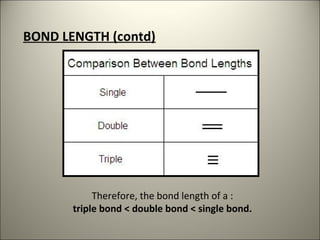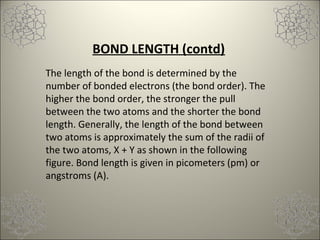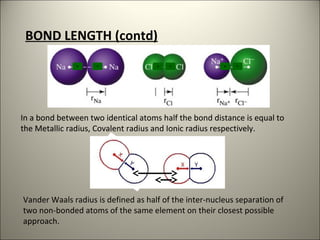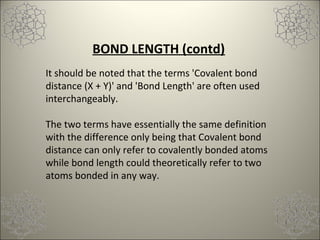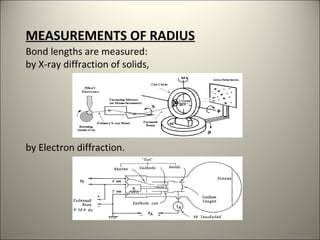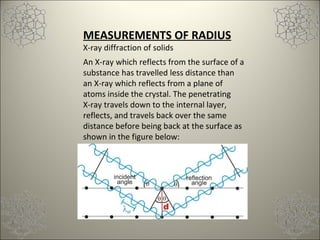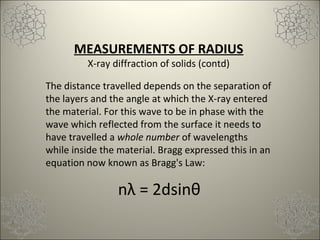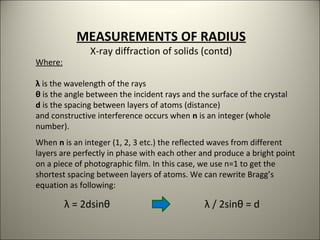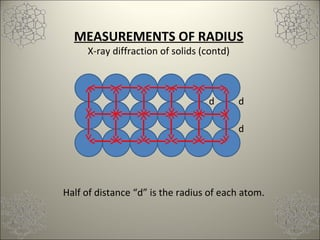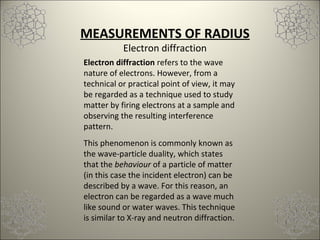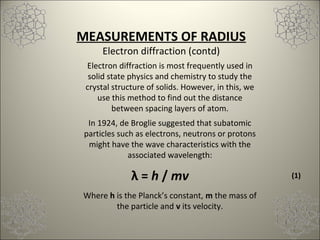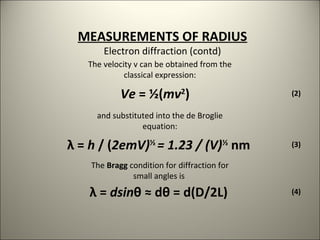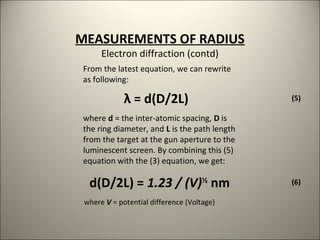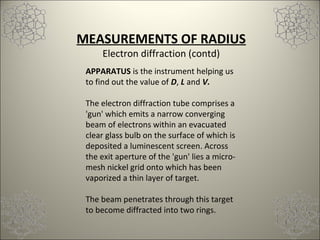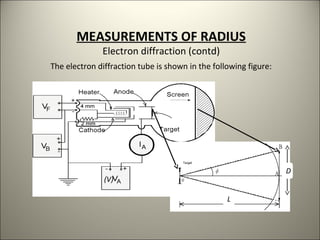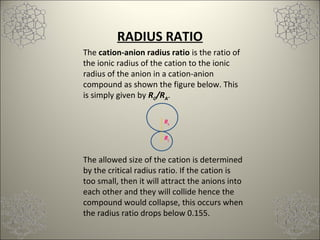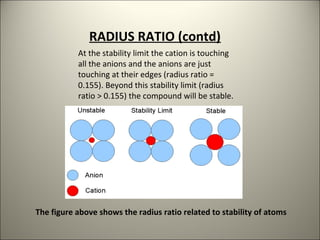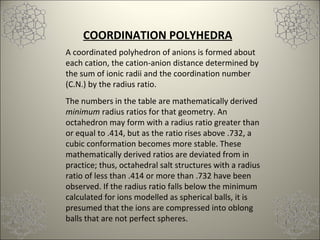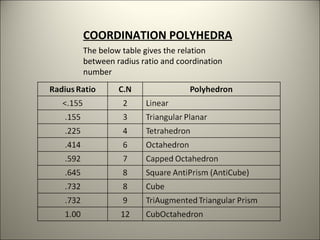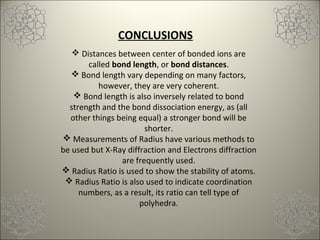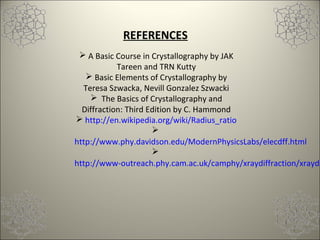1 von 25

### Bond length and measurements of radius

• 1. Bond Length and Measurements of Radius AND Radius Ratio and Co-Ordination Polyhedra
• 2. CONTENTS - Introduction - Bond Length - Measurements of Radius - Radius Ratio - Coordination Polyhedra - Conclusions - References
• 3. INTRODUCTION Distances between center of bonded ions are called bond length, or bond distances. Bond length vary depending on many factors, but in general, they are very consistent. Of course the bond orders affect bond length, but bond length of the same order for the same pair of atoms in various molecules are very consistent.
• 4. BOND LENGTH Bond length is related to bond order, when more electrons participate in bond formation the bond will get shorter. Bond length is also inversely related to bond strength and the bond dissociation energy, as (all other things being equal) a stronger bond will be shorter. A comparison of bond lengths is shown in the following table.
• 5. BOND LENGTH (contd) Therefore, the bond length of a : triple bond < double bond < single bond.
• 6. BOND LENGTH (contd) The length of the bond is determined by the number of bonded electrons (the bond order). The higher the bond order, the stronger the pull between the two atoms and the shorter the bond length. Generally, the length of the bond between two atoms is approximately the sum of the radii of the two atoms, X + Y as shown in the following figure. Bond length is given in picometers (pm) or angstroms (A).
• 7. In a bond between two identical atoms half the bond distance is equal to the Metallic radius, Covalent radius and Ionic radius respectively. X YX Y X Y Vander Waals radius is defined as half of the inter-nucleus separation of two non-bonded atoms of the same element on their closest possible approach. BOND LENGTH (contd) In a bond between two identical atoms half the bond distance is equal to the Metallic radius, Covalent radius and Ionic radius respectively.
• 8. BOND LENGTH (contd) It should be noted that the terms 'Covalent bond distance (X + Y)' and 'Bond Length' are often used interchangeably. The two terms have essentially the same definition with the difference only being that Covalent bond distance can only refer to covalently bonded atoms while bond length could theoretically refer to two atoms bonded in any way.
• 9. MEASUREMENTS OF RADIUS Bond lengths are measured: by X-ray diffraction of solids, by Electron diffraction.
• 10. MEASUREMENTS OF RADIUS X-ray diffraction of solids An X-ray which reflects from the surface of a substance has travelled less distance than an X-ray which reflects from a plane of atoms inside the crystal. The penetrating X-ray travels down to the internal layer, reflects, and travels back over the same distance before being back at the surface as shown in the figure below:
• 11. nλ = 2dsinθ MEASUREMENTS OF RADIUS X-ray diffraction of solids (contd) The distance travelled depends on the separation of the layers and the angle at which the X-ray entered the material. For this wave to be in phase with the wave which reflected from the surface it needs to have travelled a whole number of wavelengths while inside the material. Bragg expressed this in an equation now known as Bragg's Law:
• 12. λ = 2dsinθ λ / 2sinθ = d MEASUREMENTS OF RADIUS X-ray diffraction of solids (contd) Where: λ is the wavelength of the rays θ is the angle between the incident rays and the surface of the crystal d is the spacing between layers of atoms (distance) and constructive interference occurs when n is an integer (whole number). When n is an integer (1, 2, 3 etc.) the reflected waves from different layers are perfectly in phase with each other and produce a bright point on a piece of photographic film. In this case, we use n=1 to get the shortest spacing between layers of atoms. We can rewrite Bragg’s equation as following:
• 13. d Half of distance “d” is the radius of each atom. d d MEASUREMENTS OF RADIUS X-ray diffraction of solids (contd)
• 14. MEASUREMENTS OF RADIUS Electron diffraction Electron diffraction refers to the wave nature of electrons. However, from a technical or practical point of view, it may be regarded as a technique used to study matter by firing electrons at a sample and observing the resulting interference pattern. This phenomenon is commonly known as the wave-particle duality, which states that the behaviour of a particle of matter (in this case the incident electron) can be described by a wave. For this reason, an electron can be regarded as a wave much like sound or water waves. This technique is similar to X-ray and neutron diffraction.
• 15. λ = h / mv (1) MEASUREMENTS OF RADIUS Electron diffraction (contd) Electron diffraction is most frequently used in solid state physics and chemistry to study the crystal structure of solids. However, in this, we use this method to find out the distance between spacing layers of atom. In 1924, de Broglie suggested that subatomic particles such as electrons, neutrons or protons might have the wave characteristics with the associated wavelength: Where h is the Planck’s constant, m the mass of the particle and v its velocity.
• 16. Ve = ½(mv2 ) λ = h / (2emV)½ = 1.23 / (V)½ nm λ = dsinθ ≈ dθ = d(D/2L) (2) (3) (4) MEASUREMENTS OF RADIUS Electron diffraction (contd) The velocity v can be obtained from the classical expression: and substituted into the de Broglie equation: The Bragg condition for diffraction for small angles is
• 17. λ = d(D/2L) (5) d(D/2L) = 1.23 / (V)½ nm (6) MEASUREMENTS OF RADIUS Electron diffraction (contd) From the latest equation, we can rewrite as following: where d = the inter-atomic spacing, D is the ring diameter, and L is the path length from the target at the gun aperture to the luminescent screen. By combining this (5) equation with the (3) equation, we get: where V = potential difference (Voltage)
• 18. MEASUREMENTS OF RADIUS Electron diffraction (contd) APPARATUS is the instrument helping us to find out the value of D, L and V. The electron diffraction tube comprises a 'gun' which emits a narrow converging beam of electrons within an evacuated clear glass bulb on the surface of which is deposited a luminescent screen. Across the exit aperture of the 'gun' lies a micro- mesh nickel grid onto which has been vaporized a thin layer of target. The beam penetrates through this target to become diffracted into two rings.
• 19. Target D L (V) MEASUREMENTS OF RADIUS Electron diffraction (contd) The electron diffraction tube is shown in the following figure:
• 20. RC RA RADIUS RATIO The cation-anion radius ratio is the ratio of the ionic radius of the cation to the ionic radius of the anion in a cation-anion compound as shown the figure below. This is simply given by RC/RA. The allowed size of the cation is determined by the critical radius ratio. If the cation is too small, then it will attract the anions into each other and they will collide hence the compound would collapse, this occurs when the radius ratio drops below 0.155.
• 21. The figure above shows the radius ratio related to stability of atoms RADIUS RATIO (contd) At the stability limit the cation is touching all the anions and the anions are just touching at their edges (radius ratio = 0.155). Beyond this stability limit (radius ratio > 0.155) the compound will be stable.
• 22. COORDINATION POLYHEDRA A coordinated polyhedron of anions is formed about each cation, the cation-anion distance determined by the sum of ionic radii and the coordination number (C.N.) by the radius ratio. The numbers in the table are mathematically derived minimum radius ratios for that geometry. An octahedron may form with a radius ratio greater than or equal to .414, but as the ratio rises above .732, a cubic conformation becomes more stable. These mathematically derived ratios are deviated from in practice; thus, octahedral salt structures with a radius ratio of less than .414 or more than .732 have been observed. If the radius ratio falls below the minimum calculated for ions modelled as spherical balls, it is presumed that the ions are compressed into oblong balls that are not perfect spheres.
• 23. COORDINATION POLYHEDRA The below table gives the relation between radius ratio and coordination number
• 24. CONCLUSIONS  Distances between center of bonded ions are called bond length, or bond distances.  Bond length vary depending on many factors, however, they are very coherent.  Bond length is also inversely related to bond strength and the bond dissociation energy, as (all other things being equal) a stronger bond will be shorter.  Measurements of Radius have various methods to be used but X-Ray diffraction and Electrons diffraction are frequently used.  Radius Ratio is used to show the stability of atoms.  Radius Ratio is also used to indicate coordination numbers, as a result, its ratio can tell type of polyhedra.
• 25. REFERENCES  A Basic Course in Crystallography by JAK Tareen and TRN Kutty  Basic Elements of Crystallography by Teresa Szwacka, Nevill Gonzalez Szwacki  The Basics of Crystallography and Diffraction: Third Edition by C. Hammond  http://en.wikipedia.org/wiki/Radius_ratio  http://www.phy.davidson.edu/ModernPhysicsLabs/elecdff.html  http://www-outreach.phy.cam.ac.uk/camphy/xraydiffraction/xraydi